# Lesson 2: Counting Coins

Pennies - Count by 1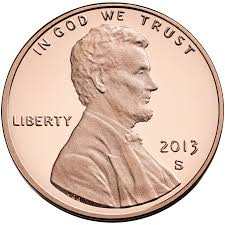=

3¢

Nickels - Count by 5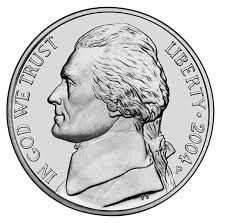=

10¢

Dimes - Count by 10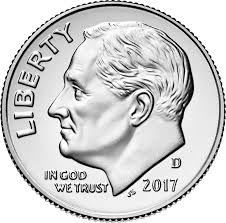=

30¢

Quarters - Count by 25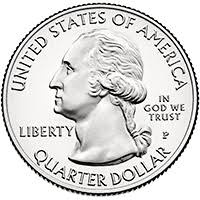=

50 ¢

Half Dollar - Count by 50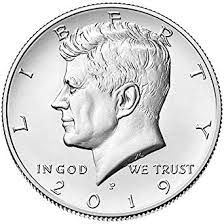=

100 ¢ or \$1.00

Gold Dollar - Count by 1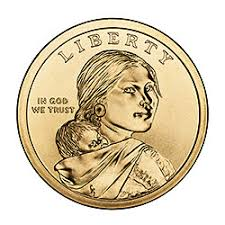=

\$3.00

Count the Coins and Write the Amount==